Universal MBQC with generalised parity-phase inter-
actions and Pauli measurements
Aleks Kissinger and John van de Wetering
April 17, 2019
We introduce a new family of models for measurement-based quantum com-
putation which are deterministic and approximately universal. The resource
states which play the role of graph states are prepared via 2-qubit gates of
the form exp(i
π
2
n
Z Z). When n = 2, these are equivalent, up to local
Cliﬀord unitaries, to graph states. However, when n > 2, their behaviour di-
verges in two important ways. First, multiple applications of the entangling
gate to a single pair of qubits produces non-trivial entanglement, and hence
multiple parallel edges between nodes play an important role in these gener-
alised graph states. Second, such a state can be used to realise deterministic,
approximately universal computation using only Pauli Z and X measurements
and feed-forward. Even though, for n > 2, the relevant resource states are no
longer stabiliser states, they admit a straightforward, graphical representation
using the ZX-calculus. Using this representation, we are able to provide a sim-
ple, graphical proof of universality. We furthermore show that for every n > 2
this family is capable of producing all Cliﬀord gates and all diagonal gates in
the n-th level of the Cliﬀord hierarchy.
Keywords: Measurement-Based Quantum Computation, ZX-calculus, Cliﬀord hierarchy
1 Introduction
Measurement-based quantum computation (MBQC) describes a family of alternatives to
the quantum circuit model, where one starts with a highly entangled resource state and
performs all computation via measurements. While individual measurements introduce
non-determinism, a deterministic quantum computation can be recovered by allowing
future operations to depend on past measurement outcomes, a concept known as feed-
forward. The most prevalent model of MBQC is the one-way model, ﬁrst described by
Briegel and Raussendorf , which makes use of cluster states or more generally graph
states and single-qubit measurements. Graph states are a family of stabiliser states that
can be described by an undirected graph, where each edge represents a pair of qubits
entangled via a controlled-Z operation. Since they are stabiliser states, any computa-
tion involving just Pauli measurements will be eﬃciently classically simulable, due to the
Gottesman-Knill theorem . In fact, any computation involving Pauli measurements can
be done in a single time-step . Hence, the entire computational power of the one-way
model comes from introducing non-stabiliser measurements to a computation.
Aleks Kissinger: aleks@cs.ru.nl, https://www.cs.ru.nl/A.Kissinger
John van de Wetering: john@vdwetering.name, http://vdwetering.name
Accepted in Quantum 2019-04-04, click title to verify 1
arXiv:1704.06504v3 [quant-ph] 16 Apr 2019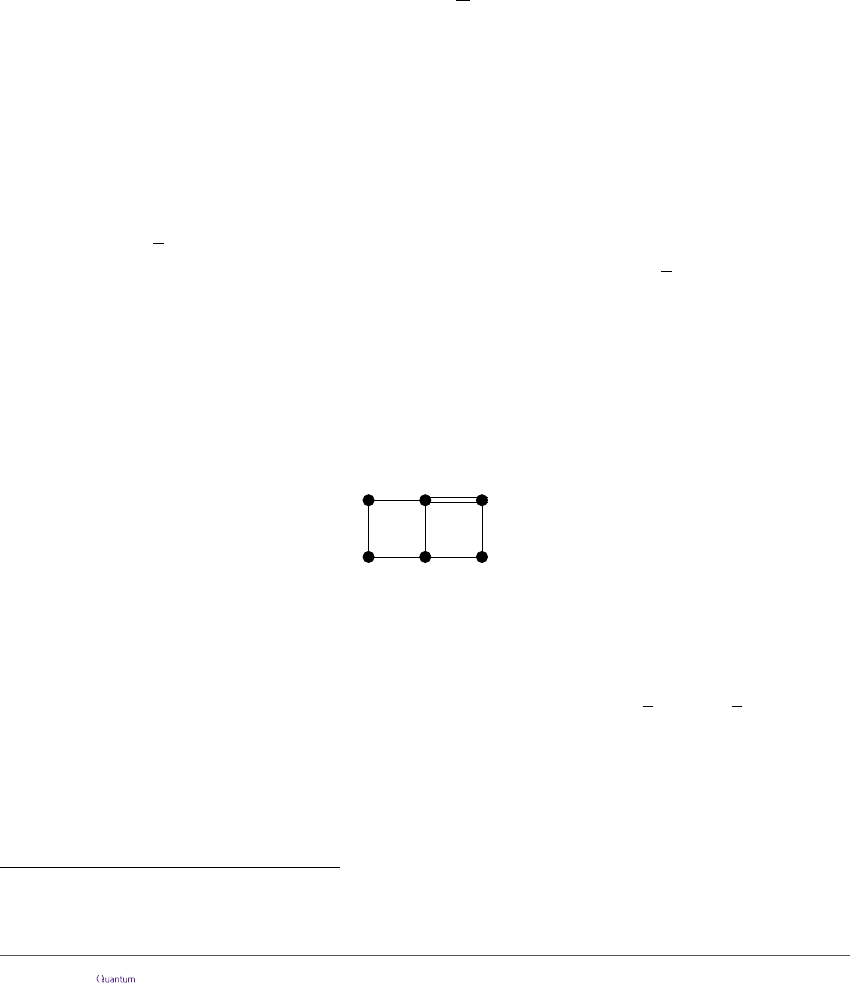It is natural to ask if we can invert this problem: is it possible to obtain universal
computation by means of a non-stabiliser resource state and just Pauli measurements?
There are several ways to achieve this. For example, one could consider resource states
which are prepared just like cluster states, but with certain qubits prepared in a |T i magic
state rather than the usual |+i state. One can also consider hypergraph states , a gen-
eralisation of graph states produced by multi-qubit n-controlled-Z operations, represented
graphically as hyper-edges. These were recently shown to admit a universal model of com-
putation using Pauli measurements and feed-forward . A diﬀerent approach was taken
in , where a resource state was created that allowed non-deterministic approximately
universal computation using just X, Y and Z measurements.
In this paper, we introduce a new family of generalisations of graph states which
admit universal deterministic computation using only Pauli X and Z measurements and
feed-forward. We call these parity-phase graph states, or P-graph states. Edges in P-graph
states represent an application of the following parity-phase gate, for some ﬁxed angle α:
P (α) = exp(i
α
2
Z Z)
We refer to this as a parity-phase gate because it introduces a relative phase of α between
its even-parity eigenstates |00i, |11i and its odd parity eigenstates |01i, |10i.
We focus on parity-phase gates because they are a popular primitive two-qubit entan-
gling gate in hardware implementations of quantum computation, such as ion trap qubits
(via Mølmer Sørensen gates [4, 14]) and, in transmon-based superconducting qubits .
At the end of Section 6, we comment brieﬂy on near-future prospects of implementing this
scheme using the latter.
When α =
π
2
, we obtain resource states which are equivalent to graph states from
the one-way model up to local Cliﬀord operations. However, if α =
π
4
, we can construct
resources which are approximately universal for quantum computation using only single-
qubit Pauli X and Z measurements and feed-forward. We call this the PPM model, for
parity-phase with Pauli measurements.
1
A key feature which distinguishes P-graph states from standard graph states is that,
unlike for controlled-Z gates, P (α)P (α) 6= 1, except in the degenerate case where α = π.
Hence it is possible, and even desirable, to consider resource states described by graphs
with multiple, parallel edges between nodes, e.g.
(1)
These parallel edges correspond to multiple applications of the entangling gate P (α) to
the adjacent qubits. For example, a doubled edge above indicates the application of
P (α)
2
= P (2α). In the graph theory literature, graphs such as (1) are sometimes referred
to as undirected multigraphs.
In the PPM model, doubled edges play a special role. Since P (
π
4
)
2
= P (
π
2
) is equiva-
lent, up to local Cliﬀord operations, to a controlled-Z gate, subgraphs of a P-graph state
containing only doubled edges behave in much the same way as traditional graph states.
However, P-graph states additionally yield the ability to selectively inject π/4 phases into
computations via nodes connected by single edges. One way to conceptualise this fact is
to consider the two-qubit gates P (π/4) as introducing ‘virtual’ magic states between pairs
1
Note that, in a previous version of this article, this model was referred to as MSPM, for Mølmer-
Sørensen with Pauli measurments.
Accepted in Quantum 2019-04-04, click title to verify 2of qubits. The phase data carried by this ‘virtual’ magic state can either be destroyed
or injected on to one of the neighbouring qubits, depending on the measurement choices,
using a method similar to e.g. Ref. .
Notably, this dichotomy gives a clean separation of the eﬃciently simulable parts of the
computation and the rest. In deriving a universal scheme for computation with P-graph
states, we will note the feed-forward is only required in the vicinity of single edges. So,
much like the case in the one-way model, the entire ‘Cliﬀord’ part of the computation can
be done in a single time step.
We make use of the ZX-calculus  for representing and reasoning about P-graph
states. This is a formalism for representing quantum states as certain tensor networks,
called ZX-diagrams, as well as a set of rules for transforming and simplifying ZX-diagrams.
In some sense, it can be regarded as an enhanced version of the stabiliser formalism, in
that any stabiliser state (or Cliﬀord circuit) can be described as a ZX-diagram, and if
two such states/circuits are equal, then one can be eﬃciently transformed into the other
by means of the ZX-calculus . However, the ZX-calculus goes beyond the stabiliser
formalism in that arbitrary linear maps between qubits can be described as ZX-diagrams.
It was recently shown that two ZX-diagrams describing the same linear map can always
be transformed into one another using an extended version of the ZX-calculus (albeit not
necessarily eﬃciently) [18, 24]. Our usage of the calculus lies somewhere between these
two extremes: we will use the ZX-calculus to eﬃciently manipulate certain well-behaved
families of non-stabiliser states.
In particular, we use a variation of the translation from graph states and measurement
patterns into ZX-diagrams given by Duncan and Perdrix  to prove the correctness of
our computation scheme. Much like in their work, and in standard approaches to MBQC,
we will demonstrate the possibility of deterministic computation by ‘pushing’ Pauli errors
forward from measurements to qubits in future, which can be corrected. However, unlike
previous work, we rely on the extra ﬂexibility of ZX-diagrams to represent non-stabilizer
correlations between qubits and develop techniques for ‘pushing’ errors through these edges
using the diagrammatic language. As we shall see, the diagrams keep track of the extra
(Cliﬀord) errors introduced by propagating errors forward, and it enables us to derive a
technique for performing Pauli and Cliﬀord corrections purely by means of single-qubit
measurement choices in the bases {X, Z}. This yields a measurement-based model which
is very ﬂexible. To give some evidence of this ﬂexibility, we show in Section 7 how to
generalise to P-graph states where a single edge denotes an application of P (
π
2
n1
). This
enables us to incorporate a familiar ‘trick’ (see e.g. [16, Section III]) into the model to
deterministically implement any diagonal gate of the n-th level of the Cliﬀord hierarchy.
Related work. To give a proof of universality, we introduce ‘hairy brickwork states’,
which are inspired by the brickwork states introduced in Ref.  for universal computation
in the one-way model. Alternatives to the one-way model have been considered, notably in
a broad range of models by Gross et al , which include a variation on graph states called
weighted graph states, whose two-qubit interactions are equivalent to P (α) for values of α
diﬀerent from π/2, up to local unitaries. However, our approach is distinct in that we use
very limited measurements and rely on the fact that P-graph states are a more powerful
resource than standard graph states, in spite of having non-maximal entanglement between
some pairs of qubits. Our scheme also has the property of the one-way model that all errors
can be corrected via feed-forward, eliminating the need for ‘trial-until-success’ strategies
used by Ref. . In Ref.  a model based on hypergraph states is constructed that only
needs Pauli measurements to become universal, but its structure is more complex than ours
and the protocols used are not deterministic. Deterministic protocols using hypergraph
Accepted in Quantum 2019-04-04, click title to verify 3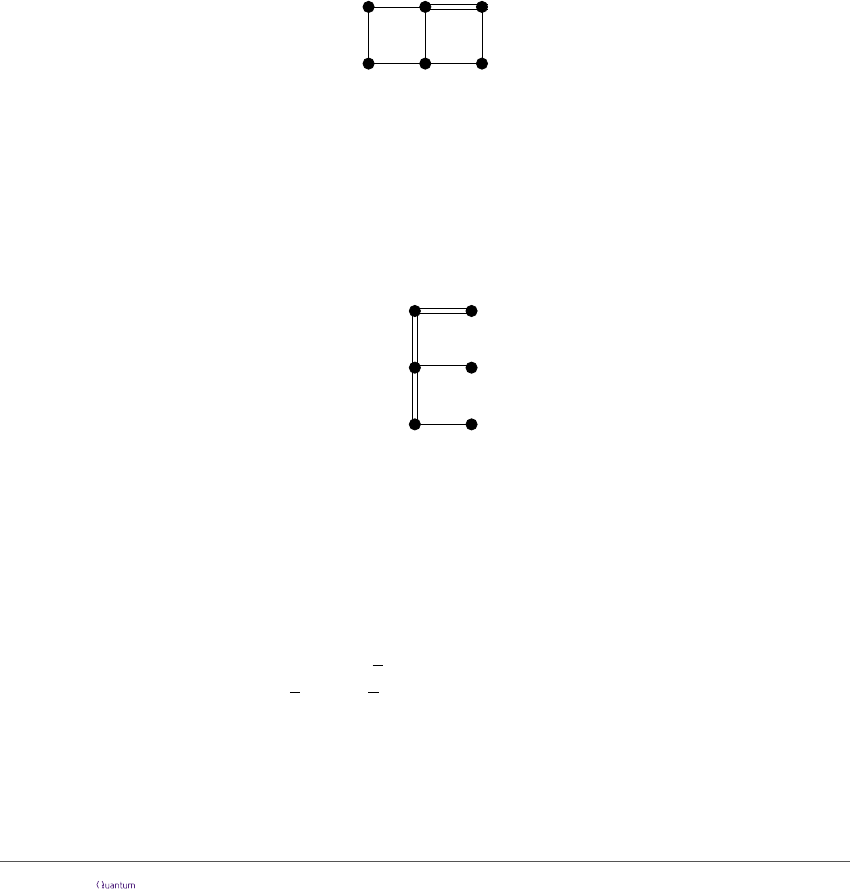states and Pauli measurements have been proposed since the appearance of this article in
preprint, namely those in Refs.  and . However, our protocol remains interesting
for several reasons. First, parity-phase interactions are typically more primitive, in that
they have simpler realisations within the gate sets of current hardware proposals. Second,
the universal gate set we produce in our model is Cliﬀord+T (or more generally, Cliﬀord
+ arbitrary diagonal Cliﬀord-hierarchy gates), as opposed to CCZ+Hadamard. While the
latter is also universal, it requires extra overhead for encoding computations in a higher-
dimensional space . Third, and perhaps most importantly, we introduce a drastically
diﬀerent methodology to existing approaches. This yields a rather ﬂexible family of models
that enable us to explore a variety of multi-qubit interactions and graph topologies. Indeed
it is a topic of active research to extend these techniques to hypergraph-based models,
where the role of the ZX-calculus is played by the recently-introduced ZH-calculus .
2 The PPM Model
A P-graph state is described by an undirected graph, where we allow multiple edges
between vertices. In practice, we will only need to consider two cases: either a single edge
or a double edge:
A single edge describes the application of an P(π/4) gate, whereas a double edge describes
the application of an P (π/2) = P (π/4)
2
gate.
A measurement pattern is a P-graph state where each node is labelled by a measurement
expression of the form b φ(a
1
, . . . , a
n
)” where b is a fresh name called the output value
and φ is a classical function from boolean variables a
1
, . . . , a
n
to a single boolean value.
In this case, we say for each a
i
that b depends on a
i
. An MS-pattern is well-founded if
there are no cyclic dependencies between variables, such as in the following pattern:
b 0
e bc d f a
a 1
c 0 d 0
These expressions do not have any explicit time-ordering, but there are restrictions on
the order in which measurements can be made, due to dependencies on prior outcomes,
i.e. feed-forward. As a matter of convention, we will typically draw earlier measurements
below later ones, i.e. ‘time’ ﬂows upward.
Computations are performed as follows:
1. A qubit is initialised in the |+i state for each vertex in a P-graph state.
2. For every edge in the graph, P (
π
4
) is applied to the two qubits at its source and
target. In particular, P (
π
2
) = P (
π
4
)
2
is applied to every pair of qubits connected by
a double edge.
3. For a qubit labelled b φ(a
1
, . . . , a
n
)”, where the values a
1
, . . . , a
n
are known,
measure in the X-basis if φ(a
1
, . . . , a
n
) = 0 and the Z-basis otherwise. In either
case, store the measurement result in b.
Accepted in Quantum 2019-04-04, click title to verify 4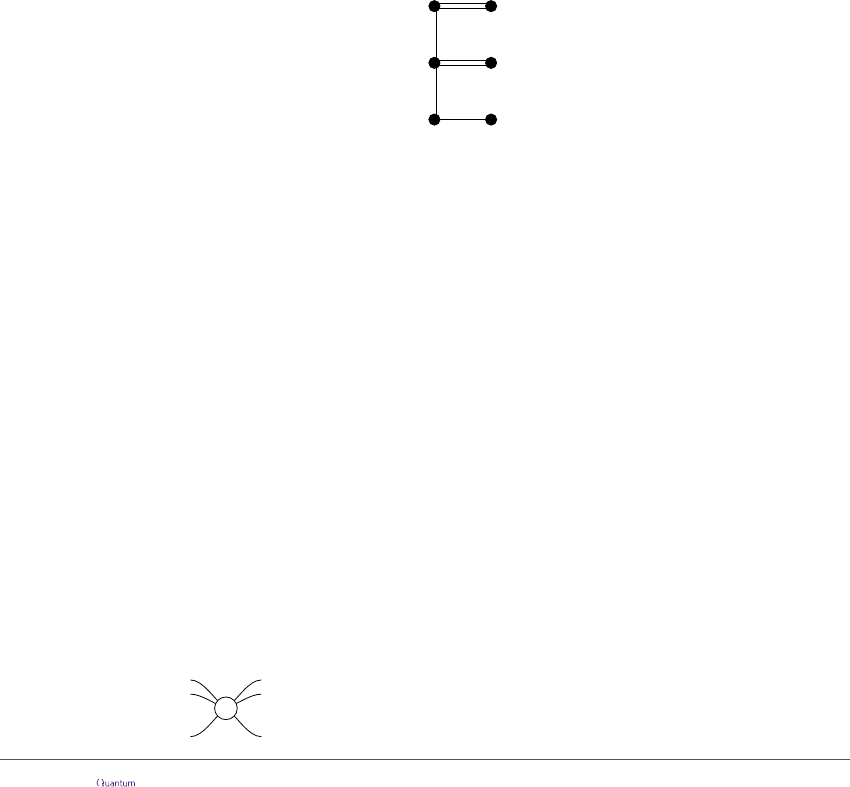4. Optionally, perform some classical post-processing on the measurement results.
As with the one-way model, the two-qubit gates all commute, so the order of application
is irrelevant, leaving only the undirected graph structure.
To show that this model is universal, we will compose smaller patterns into larger ones.
In order to do this, we give a notion of pattern fragment analogous to the notion given
for the one-way model. A pattern fragment is just like a measurement pattern, with the
exception that we additionally identify two (not necessarily disjoint) subsets of vertices
I, O V which respectively correspond to inputs and outputs. Inputs correspond to qubits
that can be in an arbitrary state, rather than the ﬁxed state |+i. Outputs correspond to
qubits which remain unmeasured after the application of the pattern-fragment.
Each vertex in I is labelled with an input error expression of the form: (z, x)
for fresh variables z and x, which capture whether a Z or X error is being fed forward into
this vertex. Unless the input vertex is also an output, the vertex will also be labelled with
a measurement expression. Measurement choice functions φ in the fragment are allowed to
depend on the input errors as well as other measurements occurring within the fragment.
Each vertex in the output set O is labelled by an expression of the form: (ζ, ξ)
consisting of a pair of classical functions ζ, ξ which again can depend on the input errors
and the results of measurements in the pattern fragment. Vertices in O are not measured
so they do not contain a measurement expression.
Here is an example of a pattern fragment where the bottom left qubit is an input, and
the top left qubit is an output:
(z, x) ; a 0
(ζ, ξ) e b x
b 1
d 0c 0
We say a pattern fragment implements a gate G if, for any input state of the form:
X
x
Z
z
|ψi, performing the pattern fragment yields X
ξ
Z
ζ
G |ψi. This extends in the natural
way to gates with multiple input/output qubits:
(X
x
1
Z
z
1
. . . X
x
n
Z
z
n
) |ψi 7→ (X
ξ
1
Z
ζ
1
. . . X
ξ
m
Z
ζ
m
)G |ψi
Composing pattern fragments then results in the composition of their associated gates,
hence it suﬃces for the sake of universality to show we can implement a universal set of
quantum gates via pattern fragments.
3 ZX-notation
To derive a universal set of measurement patterns for the PPM model, it is convenient to
use ZX-notation, which is a superset of the usual quantum circuit notation. It is used to
depict linear maps from qubits to qubits, put together in the usual way via composition
and tensor product. We will provide a brief overview. For an in-depth reference see
Ref. .
The only operations in ZX-notation are swap gates, identities, and special linear maps
called spiders. These come in two varieties, Z-spiders depicted as white dots:
α
...
...
:= |0 ···0ih0 ···0| + e
|1 ···1ih1 ···1|
Accepted in Quantum 2019-04-04, click title to verify 5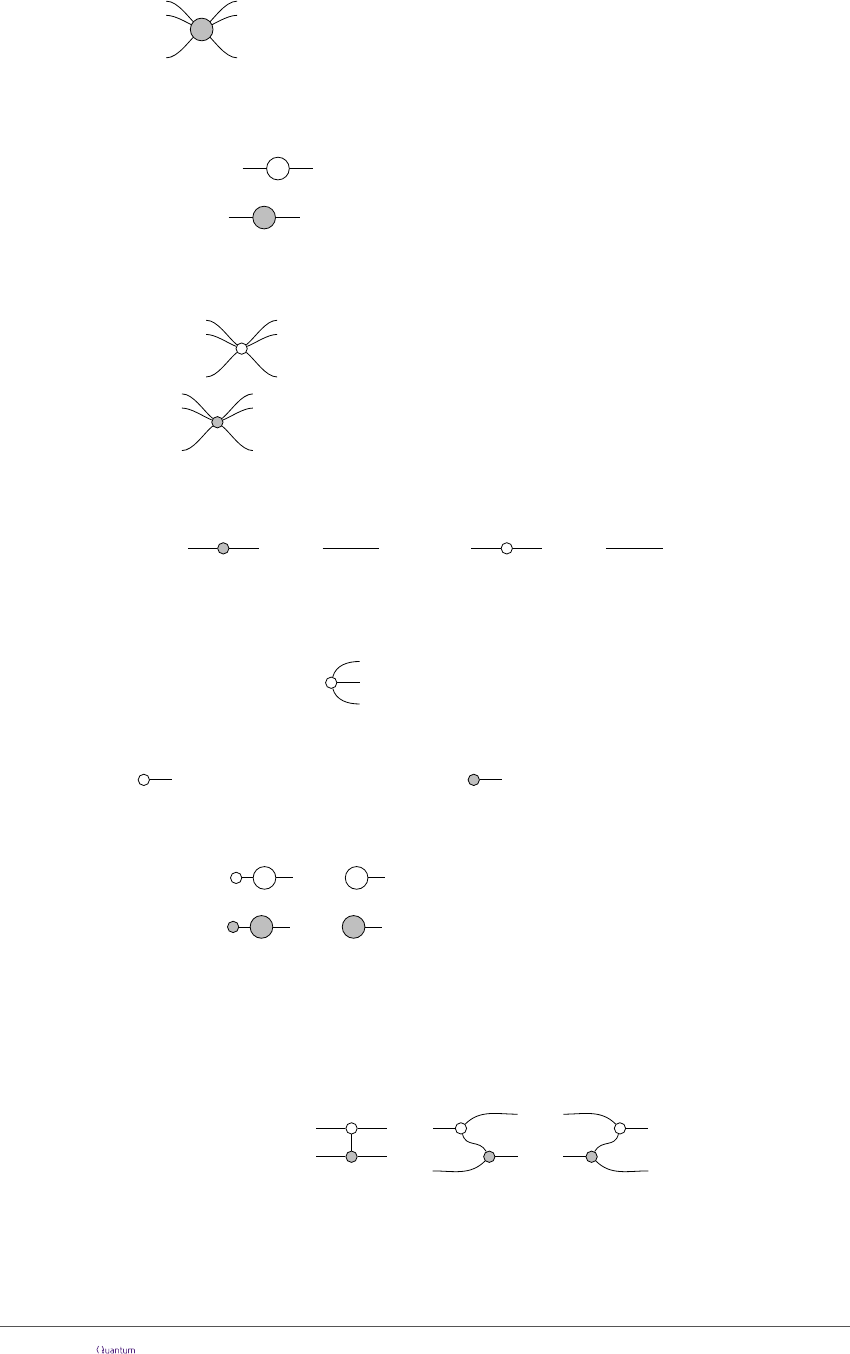and X-spiders depicted as grey dots:
α
...
...
:= |+ ···+ih+ ···+| + e
|−···−ih−···−|
A special case is when spiders have a single input and output, in which case they form
Z-phase and X-phase gates (up to a global phase):
α
= |0ih0| + e
|1ih1| = Z(α)
α
= |+ih+| + e
|−ih−| = X(α)
Note that we adopt the convention for α such that the Pauli Z and X gates are obtained
as Z(π) and X(π), respectively. Another special case is when α = 0, in which case we
omit the label:
...
...
:= |0 ···0ih0 ···0| + |1 ···1ih1 ···1|
...
...
:= |+ ···+ih+ ···+| + |−···−ih−···−|
In particular, when these phaseless spiders have single input and output they are the
identity:
= =
Phaseless spiders can be thought of as a generalisation of the GHZ state to a linear
map. Indeed the Z-spider with 0 inputs and 3 outputs is the usual GHZ state (up to
normalisation):
= |000i + |111i
Furthermore, the |0i and |+i states are just one-legged spiders:
= |0i + |1i |+i = |+i + |−i |0i (2)
The |1i and |−i states are these states followed by respectively a Z(π) and X(π) gate:
=
π π
= |0i + e
|1i |−i
π π
=
= |+i + e
|−i |1i
Note that hence forth we will ignore non-zero scalar factors and we will cease to write
instead of =, as scalar factors will not enter into our calculations.
While spiders are typically non-unitary, and hence not quantum gates, they can be
used to construct a universal family of quantum gates. We already saw Z- and X-phase
gates, while the CNOT gate can be constructed as follows:
CNOT := = =
Note that we can always reverse the direction of a wire connecting two spiders without
changing the value of the linear map, hence we can write the leftmost diagram above
without ambiguity. More generally, diagrams of spiders are less rigid than circuits. Much
Accepted in Quantum 2019-04-04, click title to verify 6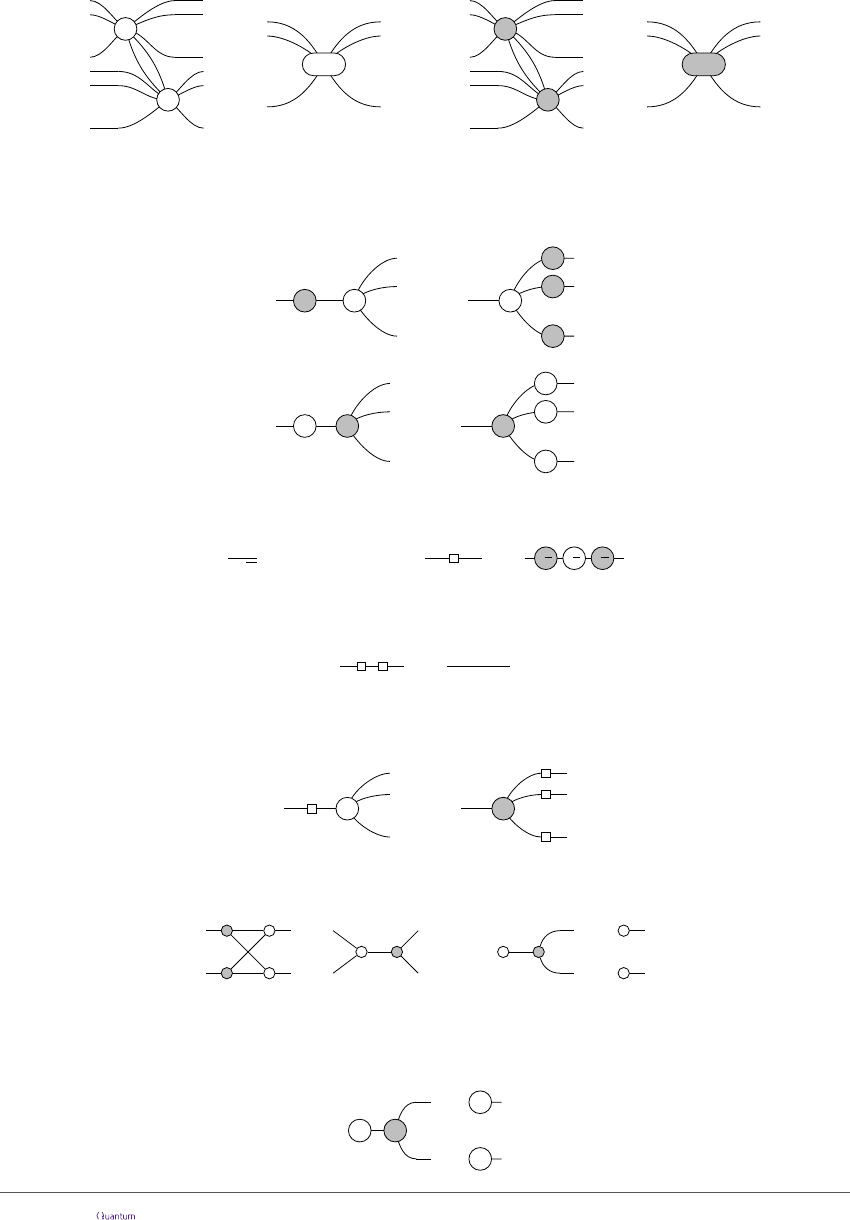like diagrammatic depictions of tensor networks (e.g. Ref. ), any two diagrams of spiders
with the same connectivity and ordering of inputs/outputs describe the same linear map.
The power of the ZX-notation comes from the set of rewrite rules associated to it,
collectively known as the ZX-calculus. The ZX-calculus has a couple of variations. We
will only need a minimal set of rules. The ﬁrst two are the spider-fusion rules, which says
adjacent spiders of the same colour fuse together, and their phases add:
β
...
...
α
...
...
=
...
...
...
α+β
β
...
...
α
...
...
=
...
...
...
α+β
This implies in particular that Z and X phase gates commute through spiders of the same
colour. Pauli Z and X gates can be pushed through spiders of the opposite colour, but
they change the sign of the angle:
απ
...
=
-α
π
...
π
π
(3)
απ
...
=
-α
π
...
π
π
(4)
We introduce a special notation for the Hadamard gate:
1
2
1 1
1 1
!
=
=
-
π
2
-
π
2
-
π
2
(5)
And of course the Hadamard is self-inverse:
=
The Hadamard gate interchanges the Z and X bases, so that it acts as a colour-changer
for spiders:
α
...
=
α
...
A ﬁnal property that we will use is the strong complementarity of the Z and X bases
of a qubit, which are captured by the following two diagrammatic rules:
= =
...
...
We will refer to these two rules, respectively, as the exchange rule and the copy rule.
Combining the rightmost equation with the other rules yields a more general version,
for any a {0, 1} and α [0, 2π):
...
...
=
α
Accepted in Quantum 2019-04-04, click title to verify 7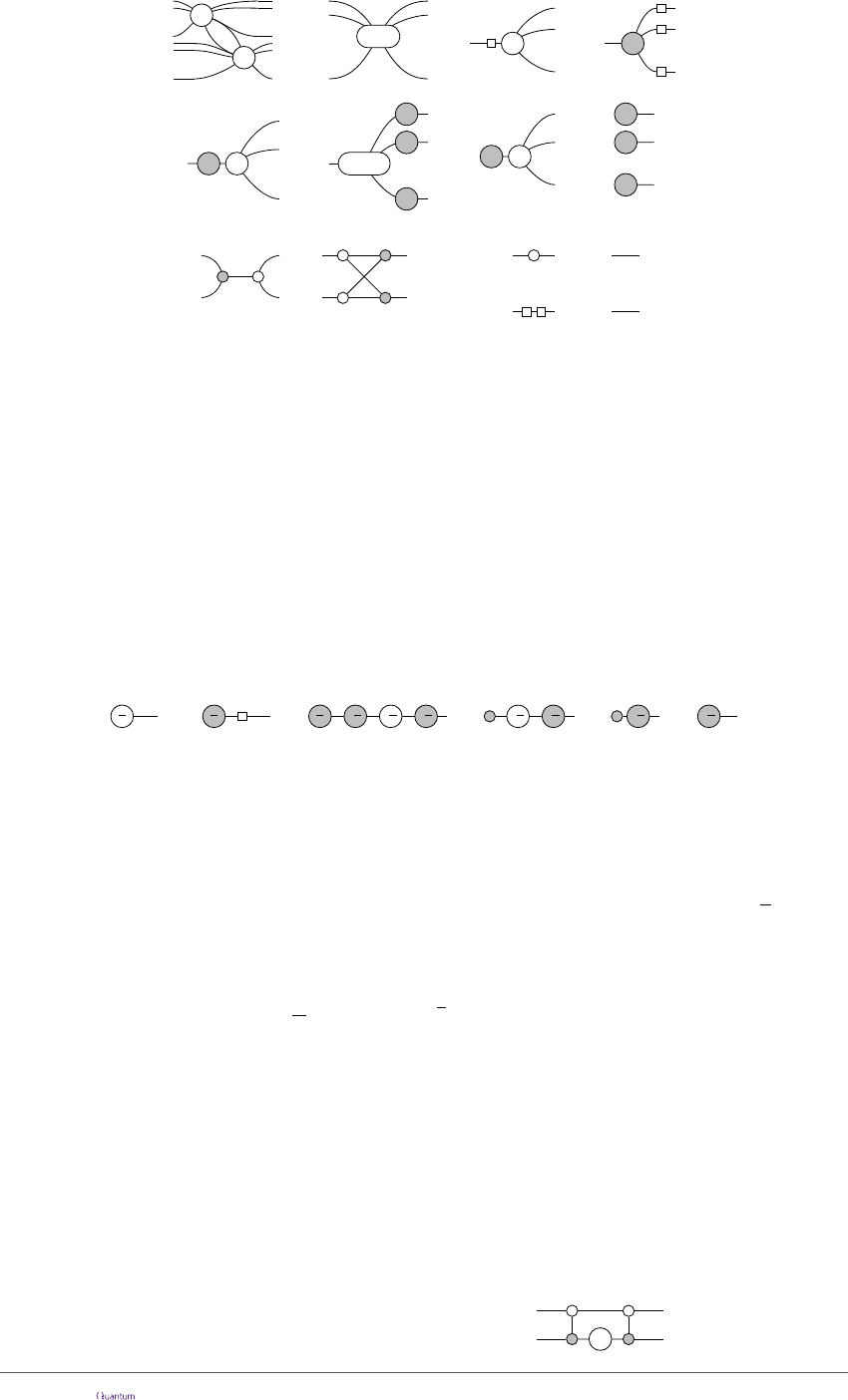β
...
...
α
...
...
=
...
...
...
α+β
(f)
(-1)
a
α
=
α
...
...
(π)
...
α
=
...
(c)
α
...
=
α
...
(h)
(i)
=
=
(hh)
(x)
=
Figure 1: The rules of the ZX-calculus: spider-(f)usion, (h)adamard, (π)-commutation, (c)opy,
e(x)change, (i)dentity, and (hh)-cancellation. Note these rules hold for all α, β [0, 2π) and a {0, 1}
and also with the colours interchanged, due to (h) and (hh).
We summarise all these rules in Figure 1. Note that an extended set of these rules
has been proven to be complete [18, 24], that is, two diagrams represent the same linear
map if and only if they can be rewritten into one another using the rules of the ZX-
calculus. In contrast, the rules of Figure 1 are only complete when restricted to the
Cliﬀord fragment .
As a demonstration of rewriting with these rules, let us derive the following equality,
which we will use throughout the paper:
π
2
=
π
2
π
2
=
-
π
2
-
π
2
-
π
2
=
-
π
2
-
π
2
=
-
π
2
=
-
π
2
(h)
(5)
(f) (π) (f)
(6)
The same equation with the colours reversed also holds.
4 PPM in the ZX-calculus
In order to express the PPM model in the ZX-calculus, we give graphical presentations for
the parity-phase gate and for Pauli measurements. Recall that P (α) = exp(i
α
2
Z Z).
It is straightforward to check that as a matrix in the computational basis, we have:
exp(i
α
2
Z Z) = e
i
α
2
1 0 0 0
0 e
0 0
0 0 e
0
0 0 0 1
If we interchange the third and fourth computational state (|10i and |11i), we obtain (up
to a global phase):
I Z(α) =
1 0 0 0
0 e
0 0
0 0 1 0
0 0 0 e
Hence we obtain the parity-phase gate by pre- and post-composing the above matrix with
CNOTs:
P (α) = CNOT(I Z(α))CNOT =
α
Accepted in Quantum 2019-04-04, click title to verify 8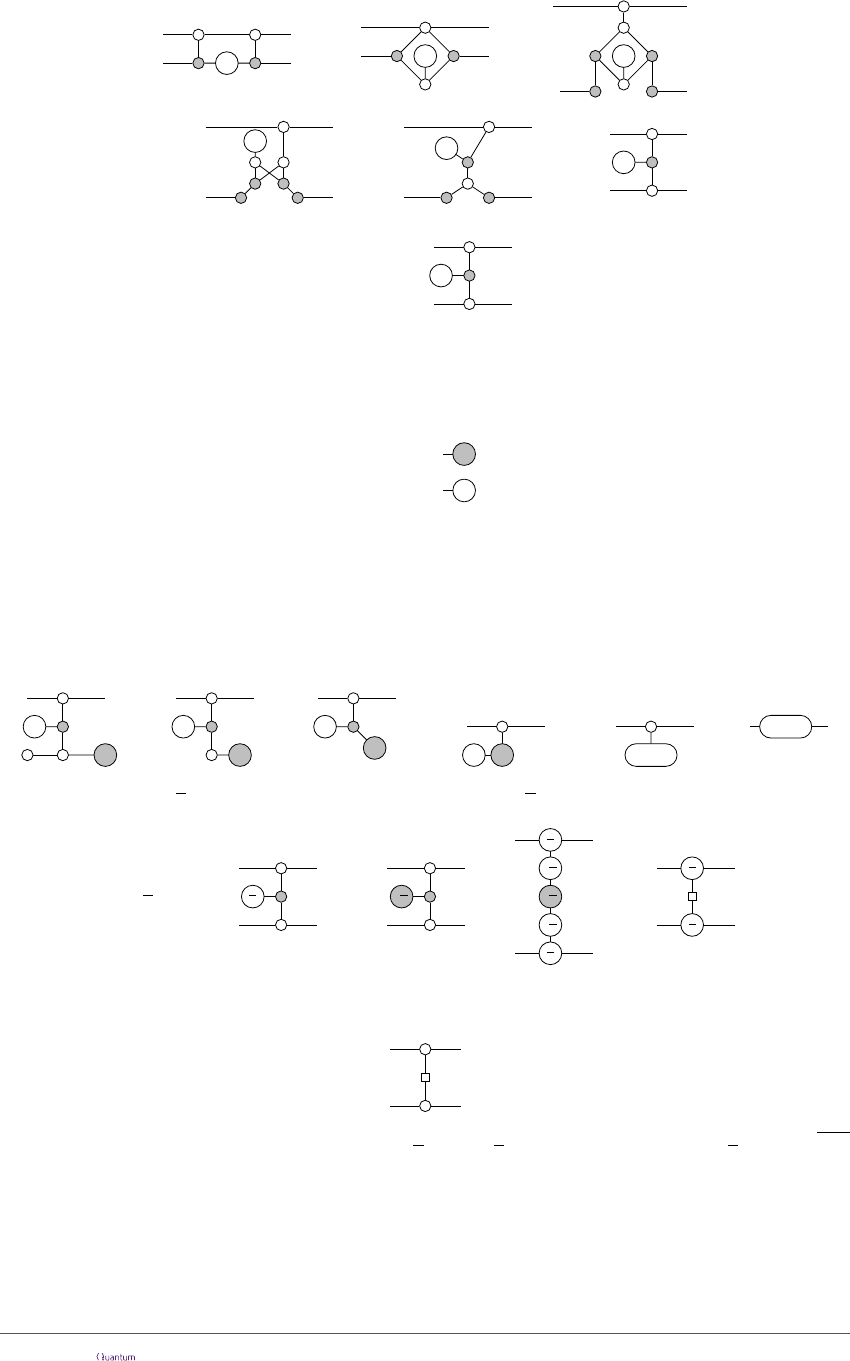This simpliﬁes as a routine calculation in the ZX-calculus (see e.g. Ref. ):
α
=
α
α
= =
α
=
α
=
α
(f) (f)
(x) (i)
Hence, we have:
P (α) =
α
(7)
The Pauli Z and X measurements non-deterministically introduce projections onto
their respective eigenstates, namely {h0|, h1|} for Z-measurements and {h+|, h−|} for X-
measurements. Because basis elements of one colour can be expressed as spiders of the
other colour, we can depict measurements as follows:
Z-measure := {
}
a∈{0,1}
X-measure := {
}
a∈{0,1}
(8)
The appearance of the ‘virtual qubit’, i.e. the state being input between the two wires
in equation (7) is quite suggestive. We can interpret this node as a magic state that is
waiting to be applied to one of its neighbouring (actual) qubits to introduce a phase. More
speciﬁcally, if we prepare the second qubit in the |+i state and then measure it in the
Z basis, we obtain a Z-phase gate Z(±α), with the sign depending on the measurement
outcome:
α
=
α
=
(-1)
a
α
(-1)
a
α
==
α α
=
(f) (i) (f) (π) (f)
If we take α =
π
2
we can rewrite the expression of P(
π
2
) in the ZX-calculus a bit further:
-
π
2
=
π
2
=
-
π
2
==
P (
π
2
)
-
π
2
π
2
-
π
2
π
2
π
2
π
2
(6)
(f)
(5)
(9)
Written in this way it is clear that the gate is equivalent, up to single-qubit Cliﬀord
unitaries to the controlled-Z gate CZ, which is represented in ZX-notation as:
Since P (α)P (β) = P (α + β) we get P (
π
2
) = P(
π
4
)
2
, so that the gate P (
π
4
) is a
CZ
gate, up to local unitaries.
Convention. From hence forth, we will typically suppress explicit references to the spider-
fusion rule (f), and assume that spiders of the same colour are (un)fused as necessary.
Similarly, we will suppress references to (i) and (hh) and simply remove 2-legged spiders
and pairs of H gates as they appear, as both are equal to the identity matrix.
Accepted in Quantum 2019-04-04, click title to verify 9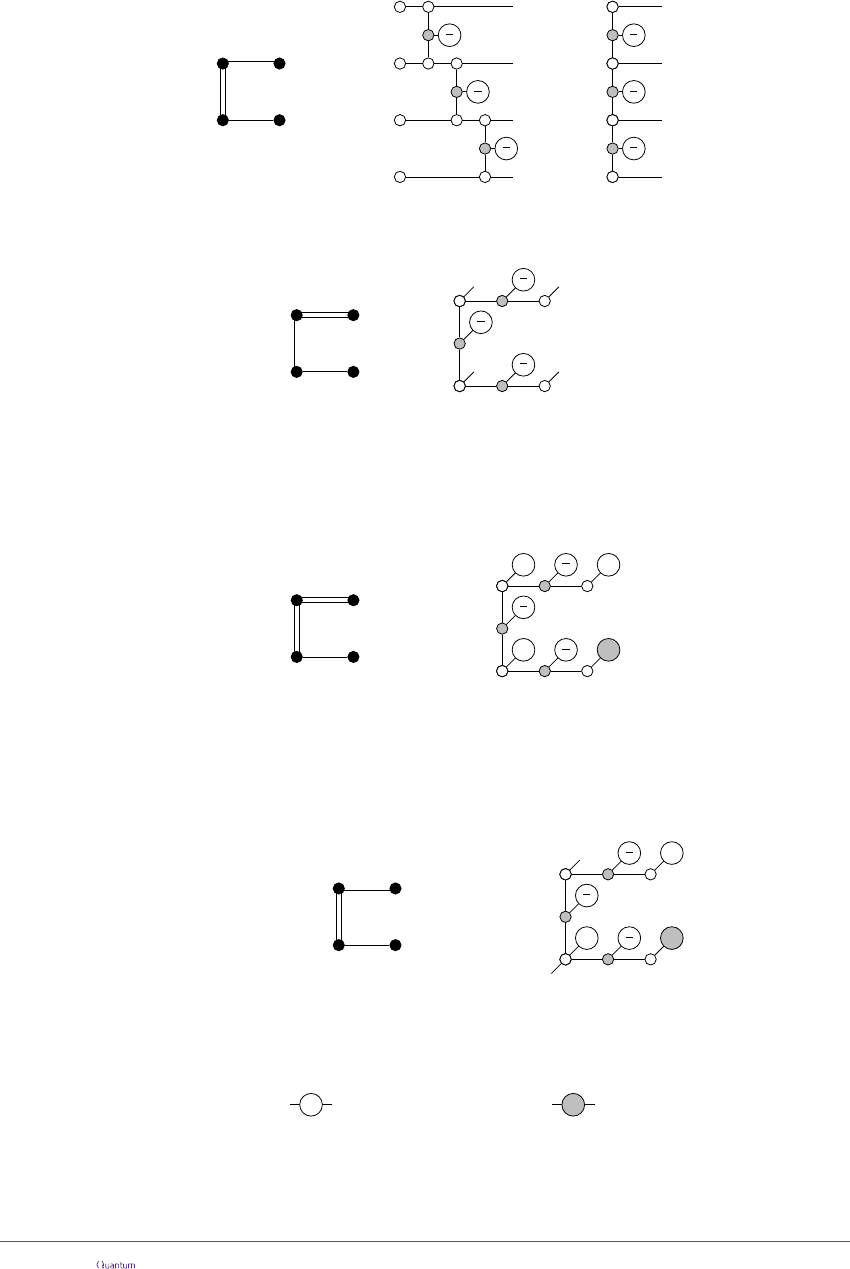We can now deﬁne the translation from P-graph states and patterns to ZX-diagrams,
which is similar in spirit to the one given in Ref.  for graph states. Qubits become
white dots with a single output and single/double edges become edges decorated by the
appropriate phases as follows:
C D
B A
7→
D
C
B
A
π
4
π
2
π
2
π
4
π
2
A
C
=
B
D
π
2
It will be convenient to deform the right-hand side to match the topology of the
associated P-graph state, in which case we can drop the qubit labels:
7→
π
4
π
2
π
2
Note that all of the wires with a free end correspond to outputs, so there is no need to
draw them exiting to the right of the diagram.
To compute the result of a measurement pattern, we post-compose with the appropriate
eﬀects {h0|, h1|} for Z-measurements and {h+|, h−|} for X-measurements using equation
(8). This enables us to write patterns (without feed-forward) as a single ZX-diagram:
7→
π
4
π
2
π
2
c 0 d 0
b 0 a 1
We could also represent the feed-forward within the diagram (e.g. by conditionally apply-
ing Hadamard gates to outputs), but for our purposes, it will be simpler just to do some
simple case-distinctions.
Finally, pattern fragments can be expressed by not measuring outputs, and adding a
new input wire for each input:
7→
π
4
π
2
π
2
(ζ, ξ) c 0
(z, x) ; b 0 a 1
(10)
In order to implement a gate G, we should show that the right-hand side above, pre-
composed with possible Pauli errors, implements G followed by some possible Pauli errors.
We can represent the possible Pauli errors as follows:
zπ
X-error :=
x∈{0,1}
z∈{0,1}
Z-error :=
(11)
Then, giving a deterministic implementation of a gate G amounts to proving that there
exists boolean functions ζ, ξ such that the following equation holds, for all values of the
boolean variables a, b, c, x, z:
Accepted in Quantum 2019-04-04, click title to verify 10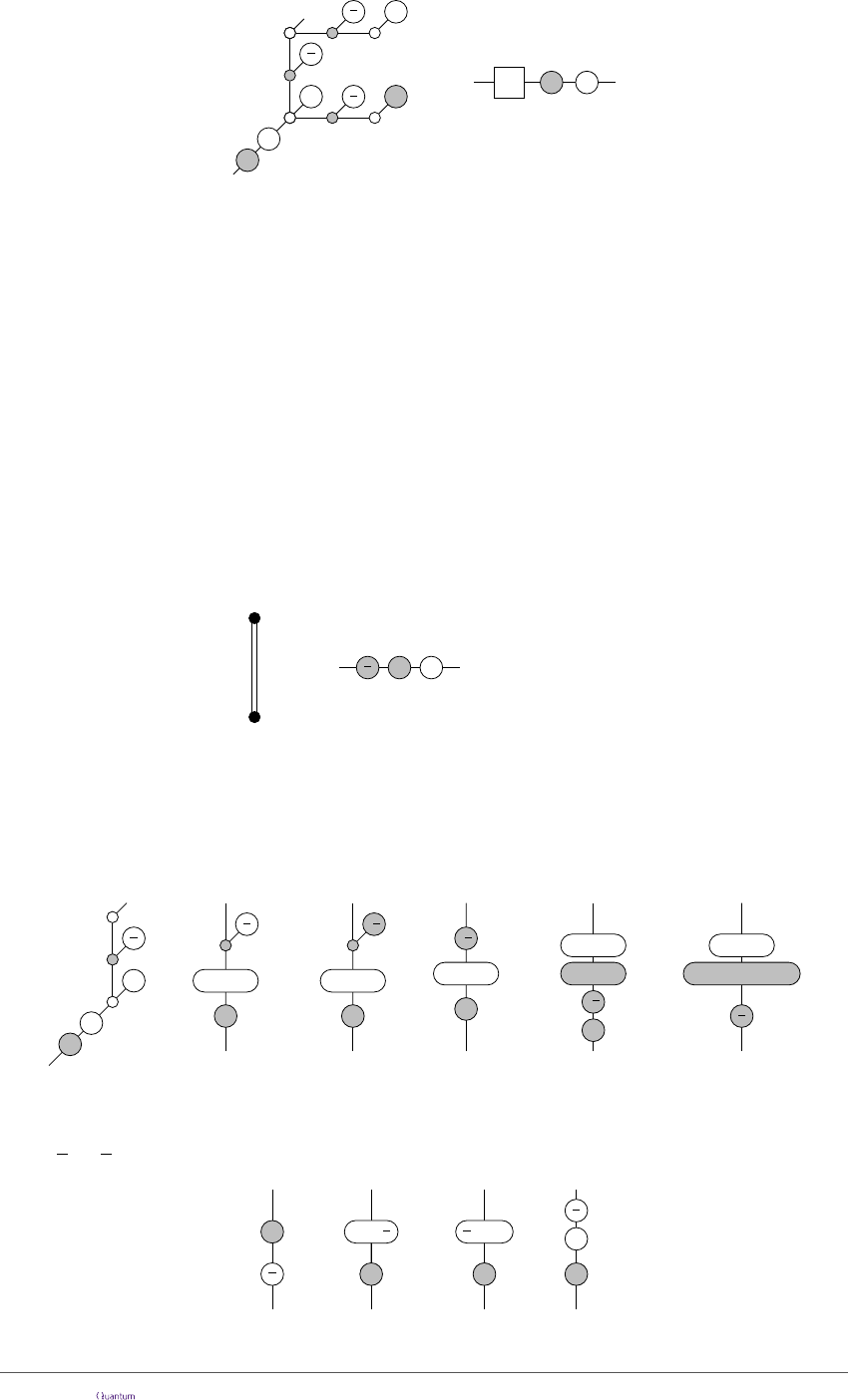π
4
π
2
π
2
zπ
=
ξπζπ
G
Remark. The colours play opposite roles in equations (8) and (11). This is a common
theme in the ZX-calculus, and comes from the fact that basis elements of one colour can
be described as spiders of the other colour (cf. equation (2)).
5 Measurement patterns for a universal set of gates
In this section, we will introduce several pattern fragments, and show using the ZX-
calculus that they deterministically implement certain quantum gates. We will start a
simple example, which uses one double-edge to implement an X(π/2) = HSH gate.
Following that, we will show that a single P-graph, with diﬀerent measurement patterns,
can implement T , H, or S gates. Similarly, we show a diﬀerent P-graph shape can be
used to selectively implement CZ or S S. These will be used in the next section to show
universality of the PPM model.
The pattern for an HSH gate is:
7→
π
2
ζπ ξπ
(z, x) ; a 0
(ζ, ξ)
where
(
ζ = z a
ξ = z a x 1
The bottom qubit is the input of the expression. We always measure it in the X basis
(a 0) which gives us a measurement result a. We record the incoming Z and X error
in the variables z and x. The resulting Z error at the end is now z a, and the X error
is z a x 1. We can show the correctness of this fragment by performing translation
(10) and reducing using the ZX-calculus:
zπ
π
2
=
(z a)π
= =
π
2
(z a x 1)π
(z a)π
π
2
-
π
2
(z a)π
=
-
π
2
(z a)π
=
(z a)π
-
π
2
(z a)π
(∗∗)(6)
Here equation (∗∗) is the standard Cliﬀord commutation law of S
X XZS
. This
commutation follows straightforwardly from (π) and the fact that, for a {0, 1}, we have
(1)
a
π
2
=
π
2
+ (mod 2π).
π
2
(-1)
a
π
2
=
(π)
π
2
=
π
2
+
=
(12)
Accepted in Quantum 2019-04-04, click title to verify 11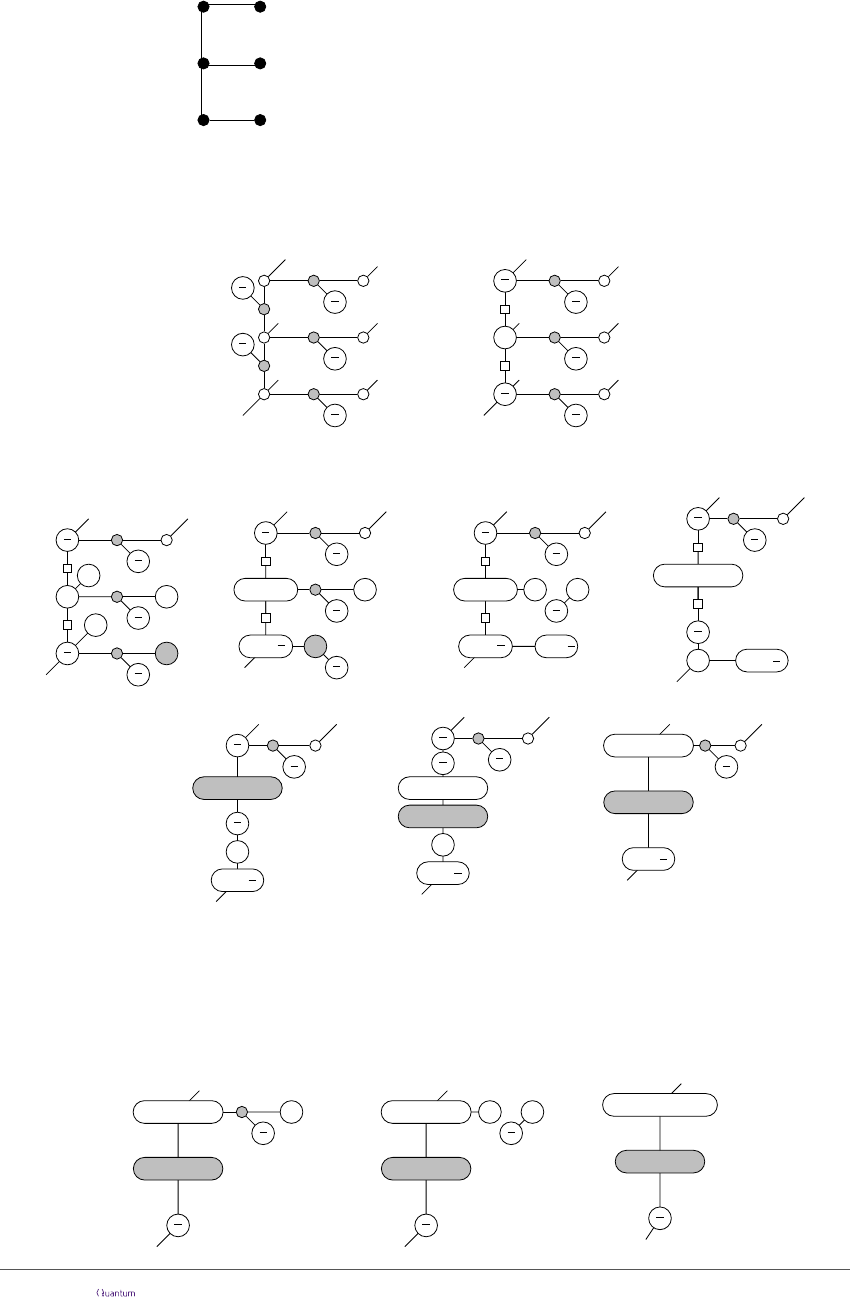We will now introduce a more versatile P-graph fragment, shaped like an ‘E’, which
can implement a variety of single-qubit gates. The ﬁrst pattern fragment in the E-shape
implements a Z(π/4) gate, i.e. a T gate:
(z, x) ; a 0
(ζ, ξ) e b x
b 1
d 0c 0
where
ζ = a c d e x (b z)(c d z 1)
ξ = c d z 1
Note that now the basis in which the qubit e is measured depends on the incoming X
error and one of the measurement results in the pattern fragment itself. Let’s translate
this to the ZX-calculus, ignoring for the moment being the Pauli errors that could be at
the beginning of the fragment:
π
2
π
π
2
π
4
π
2
=
π
2
π
4
π
2
π
2
π
2
π
2
(9)
Now we measure a, c and d in the X-basis and b in the Z-basis, each of which can introduce
a π phase of their respective colour:
π
2
(c 1)π
(-1)
a
π
2
π
4
π
2
=
π
2
(-1)
b
π
4
π
2
(c d 1)π
=
(-1)
b
π
4
π
2
(a c d)π
(c d 1)π
π
2
π
2
π
π
2
π
4
π
2
π
2
=
π
2
(c 1)π
(-1)
a
π
2
(-1)
b
π
4
π
2
π
2
(c)
(π)
=
π
2
(c d 1)π
(-1)
b
π
4
π
2
π
2
=
(h)
π
2
π
2
(-1)
b
π
4
π
2
(c d 1)π
=
π
2
(c d 1)π
(12)
(π)
()
In the step marked by () we have removed the dangling two spider diagram. We are
allowed to do this because we are ignoring scalar factors.
The goal of this pattern is to introduce a π/4 phase. We see that now we either have
π/4 or π/4. If we measure e in the X-basis, it gets cut oﬀ the main structure, but if we
measure it in the Z-basis it introduces an extra π/2 phase. So, if we got π/4 (which is the
case in the previous diagram when b = 0), we measure e in the X-basis:
π
4
π
2
(a c d)π
(c d 1)π
=
(a c d e)π
π
4
(c d 1)π
=
π
4
π
2
(a c d)π
(c d 1)π
(c)
Accepted in Quantum 2019-04-04, click title to verify 12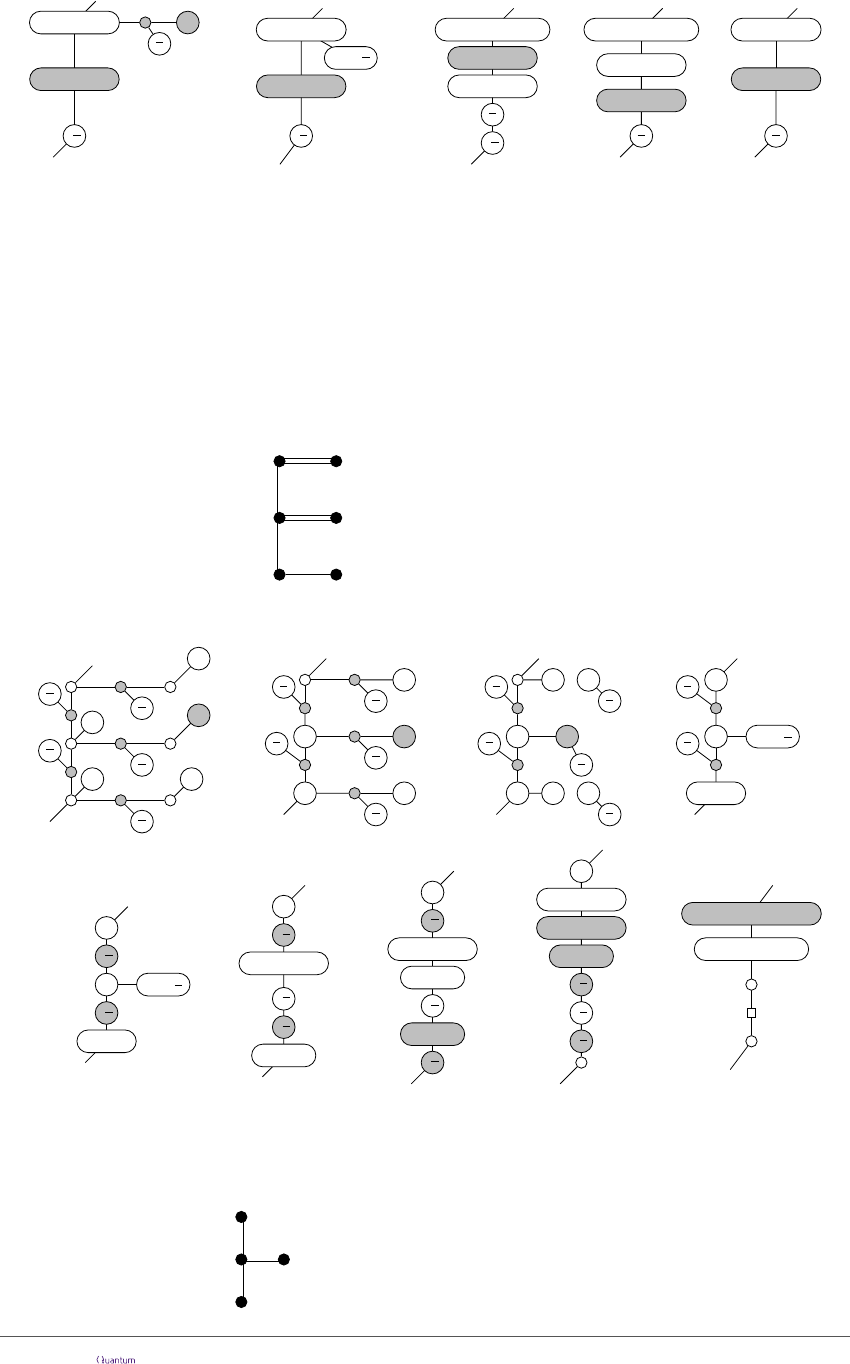and otherwise we measure e in the Z-basis:
=
-
π
4
π
2
(a c d)π
(c d 1)π
(c d 1)π
-
π
4
(a c d)π
(-1)
e
π
2
(a c d e)π
(c d 1)π
=
-
π
4
π
2
=
(a c d e)π
π
4
(c d 1)π
(c d 1)π
π
4
=
(c d 1)π
(a e 1)π
(π)
(12)
(c d 1)π
(π)
so that we indeed implement a π/4 Z-rotation with some Pauli X and Z error depending on
the measurement outcomes. In the presence of of some starting Pauli X and Z errors, the
same procedure can be done by being careful to track where the errors spread. Tracking
these errors correctly gives the pattern fragment that we started out with. If we decide to
measure e in the opposite basis (so in the Z basis when b = 0 and the X basis when b = 1),
we can implement a T
gate. Classical control determines the sign of our rotations.
Using the same pattern fragment, but with a diﬀerent set of measurements on the
‘hairs’ of the fragment we can implement some diﬀerent operators. For instance, the
following pattern fragment gives a Hadamard gate:
(z, x) ; a 0
(ζ, ξ) e 0
b 0
d 1c 0
where
(
ξ = a b c d 1
ζ = c d e 1
Note there is no feed-forward, so we can verify this in a single derivation:
π
2
π
4
π
2
π
2
π
2
-
π
2
(a b)π
-
π
2
-
π
2
(c d 1)π
=
-
π
2
-
π
2
-
π
2
(c d 1)π
(c d 1)π
(a b)π
(a b c d 1)π
=
(c d e 1)π
π
2
π
4
π
2
π
2
π
2
=
π
2
π
4
π
2
π
2
π
2
=
(c)
π
2
π
2
(a b)π
(-1)
d
π
2
=
(π)
-
π
2
-
π
2
(a b)π
(-1)
d
π
2
=
(6)
=
-
π
2
-
π
2
-
π
2
(c d 1)π
(12)
=
(a b)π
(a b)π
(12) (5)
In a similar way we can also produce an S-gate by measuring qubit e in the Z basis and
the rest in the X basis.
The following fragment implements a CZ-gate:
a 1
b 0
(z
1
, x
1
)
(ζ
2
, ξ
2
)
(ζ
1
, ξ
1
)
(z
2
, x
2
)
where
ξ
i
= x
i
ζ
1
= z
1
x